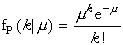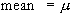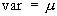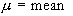Poisson Distribution

#Poisson Distribution

The Poisson Distribution represents the limit to the Binomial Distribution when events can occur continuously in time. It is useful in describing experiments in which the observed variable is a count of some sort. Some examples are: Survival times in biomedical experiments, the number of fatal traffic accidents per year in a given state, computer response time, queueing theory, the number of telephone calls per hour coming in to a switchboard, and the number of customers waiting in line at any one time. In failure analysis it models the number of failures over a period of time assuming a mean rate of failure. This can be applied to the lengths of lifetimes in service of mechanical and electrical components or the number of defects per unit of some material. In reliability theory, equipment failure often conforms to the Poisson Distribution.

The Poisson Distribution is the limit of the binomial distribution as the probability of a head becomes very small and the number of flips becomes very large. It represents the probability of k low-probability events when there are many chances for the event to occur. The only parameter of this distribution is its mean, mu. The distribution is:where k=0,1,..., and mu>0.

The equations for the mean and variance are:The equation for the parameter is:The mean must be positive.

Versions: DPL Professional, DPL Enterprise, DPL Portfolio